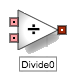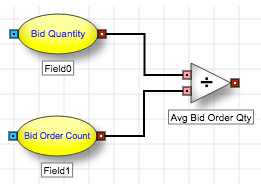# Arithmetic blocks

Arithmetic blocks

# Divide blockThe Divide block divides the first input by the second input and outputs the quotient. The order of inputs affects the outcome.

Example The Divide block divides the values of the Bid Quantity and Bid Order Count Field blocks and outputs the quotient representing the average bid order size.Note Dividing a zero by another zero will output -nan (Not A Number). In a similar manner, dividing a non-zero number by a zero will output a positive or a negative infinity. Keep in mind that these values are invalid inputs in ADL.

## Block properties

To customize the block, update the Block Properties.

Property Description
Name Name to display beneath the block on the ADL canvas